#### Electronic Transfer, Physics tutorial

Definition:

Electronic transfer is basically the determination of the thermal conductivity of electrons treated as classical particles.

Drude model:

The easiest treatment of the electrical conductivity was introduced by Drude. There are four main assumptions within the Drude model.

1) Electrons are treated as the classical particles within a free-electron approximation. Therefore, in the absence of external electromagnetic fields each and every electron is taken to move uniformly in the straight line, ignoring the interactions by other electrons and ions. In the presence of external fields each and every electron is taken to move according to the Newton's laws of motion.

2) The electrons move freely only between collisions with scattering centers. Collisions, as in kinetic theory, are instantaneous events which abruptly modify the velocity of an electron. Drude attributed them to the electrons scattering through ion cores. Though, as we will see later, this is not an exact picture of electron scattering on ordered periodic structures. A specific kind of scattering centers doesn't matter in the Drude model. An understanding of the metallic conduction can be obtained by simply supposing that there is some scattering mechanism, without inquiring too closely into just what that method might be.

3) An electron experiences a collision, resultant in an abrupt change in its velocity, by a probability per unit time 1/τ. This means that the probability of an electron undergoing a collision in any infinitesimal time interval of length 'dt' is just 'dt/τ'. The time 'τ' is thus an average time among the two consecutive scattering events. This is known as, the collision time (that is, relaxation time); it plays a basic role in the theory of metallic conduction. It follows from this supposition that an electron picked at arbitrary at a given moment will, on the average, travel for a time 't' before its next collision. The relaxation time 't' is taken to be independent of the electron's position and velocity.

4) Electrons are supposed to accomplish thermal equilibrium by their surroundings only via collisions. Such collisions are supposed to maintain local thermo-dynamic equilibrium in a particularly simple manner: immediately after each and every collision an electron is taken to emerge by a velocity that is not associated to its velocity just before the collision, however arbitrarily directed and with a speed suitable to the temperature prevailing at the place where the collision take place.

Now we take the application of the Drude model for electrical conductivity in a metal. According to Ohm's law, the current 'I' flowing in a wire (figure shown below) is proportional to the potential drop V = V2 - V1 all along the wire: V = IR, here R, the resistance of the wire, depends on its dimensions. This is much more convenient to state the Ohm's law in a form that is independent of the dimensions of the wire as such factors are irrelevant to the fundamental physics of the conduction. We state the conductivity that is the proportionality constant between the current density 'j' and the electric field 'E' at a point in the metal: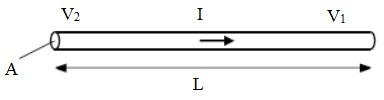j = σ E

The current density 'j' is a vector, parallel to the flow of charge, whose magnitude is the amount of charge per unit time crossing the unit area perpendicular to the flow. Therefore if a uniform current 'I' flows via a wire of length 'L' and cross-sectional area 'A', the current density will be j = I/A. As the potential drop all along the wire will be V = EL. The above equation provides I/A = σV/L, and therefore R = L/σA = ρ L/A, here we have introduced the resistivity ρ = 1/σ. Dissimilar R, σ and ρ is a property of the material, as it doesn't depend on the size and shape. Now we wish for to deduce 'σ' in terms of the microscopic properties by using the Drude model. If 'n' electrons per unit volume all move having velocity 'v', then the current density they give rise to will be parallel to 'v'. Moreover, in a time 'dt' the electrons will advance through a distance 'vdt' in the direction of 'v', so that n(vdt)A electrons will cross the area A perpendicular to the direction of flow. As each and every electron carries a charge -e, the charge crossing A in the time 'dt' will be - nevAdt and therefore the current density is:

j = nev

At any point in a metal, electrons are for all time moving in a variety of directions having a variety of thermal energies. The total current density is therefore represented by the above equation; here 'v' is the average electronic velocity or drift velocity. In the absence of an electric field, electrons are as possible to be moving in any one direction as in any other, 'v' averages to zero, and, as expected, there is no total electric current density. In the presence of a field 'E', though, there will be a drift velocity directed opposite to the field (that is, the electronic charge being negative), that we can calculate as follows. Consider a typical electron at time zero. Assume 't' be the time elapsed as its last collision. Its velocity at time zero will be its velocity Vo instantly after that collision plus the additional velocity -eE τ/m it has then acquired. As we suppose that an electron comes out from a collision in an arbitrary direction, there will be no contribution from vo to the average electronic velocity, which should thus be given completely by the average of -eE τ/m. Though, the average of 't' is the relaxation time 'τ'. Thus,

v = - eE τ/m

j = - (ne2 τ/m) E

The conductivity is, thus, represented by:

σ = ne2τ/m

We notice that the conductivity is proportional to the density of electrons that is not surprising as the higher the number of carriers, the more the current density. The conductivity is inversely proportional to the mass as the mass find out the acceleration of an electron in the electric field. The proportionality to 'τ' follows as 'τ' is the time between the two consecutive collisions. Thus, the larger 'τ' is, the more time for electron to be accelerated between the collisions and as a result the larger the drift velocity.

The values of relaxation time can be obtained from the measured values of electrical conductivity. For illustration at room temperature the resistivity of most of the metals lies in the range of 1-10 µ Ωcm. The corresponding relaxation time is of the order of 10-14s.

In this explanation of electrical conductivity we treated electrons on a classical basis. How are the results altered if the quantum mechanics is taken into account? Let us refer to the figure shown below. In the absence of an electric field, the Fermi sphere is cantered at the origin (first part of the figure). The various electrons are all moving - some at extremely high speeds - and they carry individual currents. However the net current of the system is zero, because, for each and every electron at velocity 'v' there exists other electron having velocity '-v', and the sum of their two currents is zero. Therefore the total current vanishes due to the pair wise cancellation of the electron currents.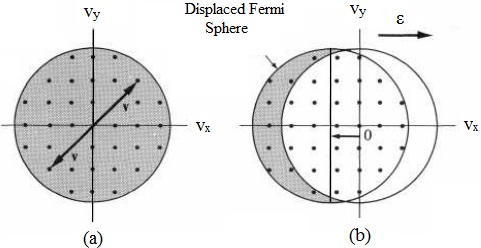The condition changes whenever a field is applied. If the field is in the positive x-direction, each and every electron obtains a drift velocity, as represented by the equation v = - eE τ/m. Therefore the whole Fermi sphere is relocated to the left, as represented in the second part of the figure above. However the displacement is extremely small, and though the huge majority of the electrons still cancel one other pair-wise, some electrons - in the shaded crescent in the figure -remain uncompensated. It is these electrons that generate the observed current.

The extremely small displacement is due to a relatively small drift velocity. If we suppose that the electric field is 0.1V/cm, we get the drift velocity of 1cm/s, which is by 8 orders in magnitude smaller the Fermi velocity of electrons.

Let us approximate the current density: The fraction of electrons that remain uncompensated is around v/vF. The concentration of such electrons is thus n(v/vF), and since each and every electron consists of a velocity of around vF, the current density is represented by:

j = -en(v/vF)vF = -nev

This is the similar expression we obtained before. Thus, generally the conductivity is deduced by the similar formula (σ = ne2τ/m). Though, the real picture of electrical conduction is therefore quite dissimilar from the classical one. In the classical picture, we supposed that the current is carried equally via all electrons, each moving by an extremely small drift velocity 'v'. In the quantum-mechanical picture the current is taken only by extremely small fraction of electrons, all moving by the Fermi velocity. The relaxation time is found out only by electrons at the Fermi surface, as only these electrons can contribute to the transport properties. Both the approaches lead to the similar result; however the subsequent is theoretically the more accurate.

As only electrons at Fermi surface contribute to the conductance, we can state the mean free path of electrons as l = τvF. We can make an estimation of the mean free path for metal at room temperature. This estimate provides a value of 100Å. Therefore it is of the order of a few tens interatomic distances. At low temperatures for extremely pure metals the mean free path can be made as high as a few cm.

The origin of collision time:

We observe that between the two collisions, the electron travels a distance of more than 20 times the interatomic distance. This is much bigger than one would anticipate if the electron in actuality did collide by the ions whenever it passed them. This paradox can be described only by using quantum theories according to which an electron consists of a wave character. This is well known from the theory of wave propagation in the periodic structures that, whenever a wave passes via a periodic lattice, it carries on propagating indefinitely without scattering. The effect of the atoms in the lattice is to absorb energy from the wave and radiate it back, in such a way that the total result is that the wave continues without modification in either direction or intensity.

Thus we observe that, if the ions makes a perfect lattice, there is no collision at all - that is, l = ∞ - and therefore τ = ∞, that in turn leads to the infinite conductivity. This has been shown, though, that the observed l is around 102 A. The finiteness of 'σ' should therefore be due to the deviation of the lattice from perfect periodicity; this occurs either because of (i) thermal vibration of the ions, or due to (ii) the presence of imperfections or the foreign impurities.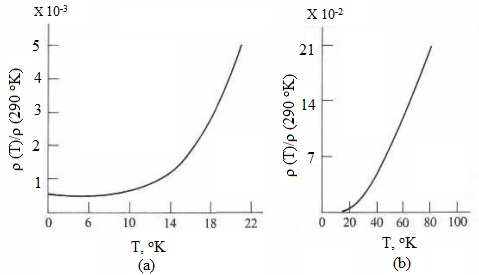In order to take their contribution we look at the temperature dependence of the electrical conductivity. The electrical conductivity of a metal differs by temperature in a characteristic way. This variation is generally illustrated in terms of the behavior of the resistivity 'ρ' versus 'T'.

The figure above represents the observed curve for Na. At T ~ 0°K, ρ consists of a small constant value; above that, ρ rises with T, slowly at first, but afterward ρ increases linearly with T. The linear behavior carries on essentially till the melting point is reached. This pattern is followed by most of the metals, and generally room temperature falls into the linear range. We want to describe this behavior of in terms of the Drude formula. Remembering that ρ = σ-1, we have

ρ = m/ne2τ

As we have illustrated earlier 1/τ that enters the above equation, is the probability of the electron scattering per unit time. Therefore, if τ = 10-14 s, then the electron undergoes 1014 collisions in one second. We found out that the electron experiences collisions only because the lattice is not perfectly regular. We categorize the deviations from a perfect lattice into two classes.

1) Lattice vibrations (that is, phonons) of the ions around their equilibrium position due to the thermal excitation of the ions.

2) All the static imperfections, like impurities or crystal defects. Of this latter group we should take impurities as an illustration.

The total probability for an electron to be scattered in a unit time is the total sum of the probabilities of scattering through phonons and by impurities. This is because such two methods are supposed to act independently. Thus we might write:

1/τ = 1/τi + 1/τph

Here the first term on the right is due to the impurities and the second is due to the phonons. The scattering via impurities is basically independent of temperature, while the scattering through phonons is temperature dependent as the number of phonons rises with temperature. If the above equation is replaced into (ρ = m/ne2τ), we readily get:

ρ = ρi + ρph = m/ne2τi + m/ne2τph

We notice that 'ρ' has split into two terms. A term ρi due to scattering via impurities that is independent of 'T', is termed as the residual resistivity. The other term ρph(T) is due to the scattering by phonons; therefore it is temperature dependent. At times it is termed as the lattice resistivity.

At extremely low 'T', scattering by phonons is negligible as the amplitudes of oscillation are very small; in that region τph → ∞, ρph → 0, and therefore ρ = ρi is a constant. This is in agreement with the above figure. As 'T' increases, scattering through phonons becomes more efficient, and ρph(T) increases; this is why ρ increases. If 'T' becomes adequately large, scattering through phonons dominates and ρ ~ ρph(T). The statement that ρ can be split into two parts, is termed as the Matthiessen rule. This rule is personified in the above equation.

In common, the Matthiessen rule predicts that if there are two distinguishable sources of scattering (such as in the case above: phonons and impurities) the resistivity is the sum of the resistivities due to the first and the second method of scattering. The Matthiessen rule is sort of empirical observation that can be employed for a qualitative understanding of the contribution from different scattering methods. Though, one should for all time bear in mind the possibility a failure of this rule. In particular, in the case when the relaxation time based on the wave vector k, the Matthiessen rule becomes unacceptable.

Now let us derive approximate expressions for τi and τph by using arguments from the kinetic theory of gases. Consider first the collision of electrons having impurities. We write:

τi = li/vF

Here li is the mean free path for collision having impurities. In order to determine the mean free path we shall suppose, for simplicity, that the collision is of the hard-spheres (that is, billiard-ball) kind and introduce the scattering cross section of an impurity Σi, that is the area an impurity atom presents to the incident electron. Then, we can argue that the product of the mean free path and the cross section of impurity, liΣi, are equivalent to the average volume per impurity, 1/ni, where ni is the impurity concentration, that is,

liΣi = 1/ni,

And thus,

li = 1/niΣi

The scattering cross section Σi is of the similar magnitude as the real geometrical area of the impurity atom. That is, that Σi ~ lÅ2. Computations of the exact value of Σi need quantum scattering theory. By replacing the equation (li = 1/niΣi) and (τi = li/vF) into [ρ = ρi + ρph = m/ne2τi + m/ne2τph, we get:]

ρi = (mvF/ne2) niΣi

As expected, ρi is proportional to ni that is the concentration of impurities.

Computing ρph is much harder, however equations identical to (τi = li/vF) and (li = 1/niΣi) still hold. In particular, one might write:

lph = 1/naΣa

Here, na is the concentration of the host atoms in the lattice, and Σa is the scattering cross section per atom. We must note here that Σa consists of no relation to the geometrical cross section of the atom. Instead it is the area represented by the thermally fluctuating atom to the passing electron. Assume that the distance of deviation from equilibrium is x, then the average scattering cross section is:

Σa ∝ <x2>

Here <x2> is the average of x2. We can simply approximate this value at high temperatures, if the classical approach is valid. As the ion is a harmonic oscillator, the value <x2> is proportional to the average of its potential energy is equivalent to half the total energy. Therefore,

Σa ∝ <x2> ∝ (kB/2C)T

Here, C is interatomic force constant introduced previous and we utilized the formula for the energy of a classical oscillator. We observe thus that at high temperatures the resistivity is linear in 'T',

ρph ∝ (mvFna/ne2) (kB/2C) T

That is in agreement by the experiment.

In low-temperature range the lattice resistivity differs by temperature in a different manner. By employing the Debye model at low temperature range one can determine that ρph ~ T5

Thermal conductivity:

If the ends of a metallic wire are at different temperatures, heat flows from the hot to cold end. The fundamental experimental fact is that the heat current density, jQ, that is, the amount of thermal energy crossing a unit area per unit time is proportional to the temperature gradient,

jQ = -K (dT/dx)

Here, 'K' is the thermal conductivity. In insulators, heat is taken out completely by phonons, however in metals heat might be transported through both phonons and electrons. The thermal conductivity 'K' is thus equivalent to the sum of the two contributions,

K = Ke + Kph

Here, Ke and Kph refer to electrons and phonons, correspondingly. In most of the metals, the contribution of electrons greatly goes beyond that of the phonons, because of the huge concentration of electrons, usually Ke ~ 102 Kph.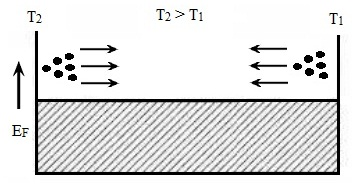The physical method by which heat conduction occurs through electrons is described in the figure above. Electrons at the hot end (that is, to the left) travel in all directions, however a certain fraction travel to the right and carry energy to the cold end. Likewise, a certain fraction of the electrons at the cold end (that is, on the right) travel to the left, and carry energy to the hot end. Since on the average electrons at the hot end are more energetic than such on the right, a total energy is transported to the right, resultant in a current of heat. Note that heat is transported completely through electrons having the Fermi energy, as those well beneath this energy cancel one other's contributions.

To compute the thermal conductivity 'K' quantitatively, we make use of the formula K = (1/3)CelvFl, here Cel is the electronic specific heat per unit volume, 'v' is the Fermi velocity of electrons, 'l' is the mean free path of electrons at the Fermi energy. By employing the expression for the heat capacity derived earlier, we determine:

K = (1/3)[(π2/2)n(KB2T/EF)]vFl

Noting that EF = 1/2mvF2 and that l/vF = τ, we can simplify this expression for 'K' to

K = π2nKB2τT/3m

that deduces the thermal conductivity in terms of the electronic properties of the metal. Most of the parameters appearing in the expression for 'K' were as well comprised in the expression for electrical conductivity 'σ'. Recalling that σ = ne2τ/m, we determine:

K/σ = (1/3)(πKB/e)2 T = LT

We notice here that the ratio of the thermal conductivity to the electrical conductivity is directly proportional to the temperature. This is termed as the Wiedemann-Franz law. The constant of proportionality 'L' that is termed as the Lorentz number is independent of the particular metal. This depends only on the universal constants kB and e, must be similar for all metals. Its numerical value is 2.45 x 10-8 WΩ/K2. This conclusion recommends that the electrical and thermal conductivities are intimately associated that is to be expected, as both electrical and thermal current are carried through the similar agent: electrons.

Motion in a magnetic field: cyclotron resonance and Hall Effect

The application of a magnetic field to a metal gives mount to some interesting phenomena due to the conduction electrons. The cyclotron resonance and the Hall Effect are two that we consider.

Cyclotron resonance:

Whenever a magnetic field is applied to the metal the Lorentz force F = -e [E+(v × B)] acts on each and every electron. For a perfect metal in the absence of electric field the equation of motion is:

mdv/dt = - ev x B

If the magnetic field lies all along the z-direction this outcome in:

dvx/dt = - ωCvy

dvy/dt = ωCvx

Here, ωC = eB/m

is the cyclotron frequency in the SI system of units (in CGS ωC = eB/mc). For magnetic fields of the order of a few kG the cyclotron frequencies lie in the range of some GHz. For illustration for B = 1kG, the cyclotron frequency is νc = ωC/2π = 2.8 GHz. Thus, the magnetic field causes electrons to move in the counterclockwise circular fashion by the cyclotron frequency in a plane normal to the field.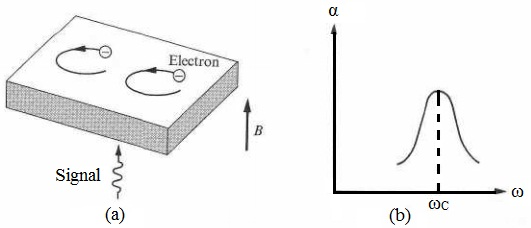Assume that now that an electromagnetic signal is passed via the slab in a direction parallel to B, as shown in the figure above. The electric field of the signal acts on the electrons, and some of the energy in the signal is absorbed. The rate of absorption is highest if the frequency of the signal is precisely equivalent to the frequency of the cyclotron, that is,

ω = ωC

This is so because, if this condition holds true, each and every electron moves synchronously by the wave all through the cycle, and thus the absorption carries on all via the cycle. Therefore, the equation (ω = ωC) is the condition for cyclotron resonance. On the other hand, if equation (ω = ωC) is not satisfied, the electron is in phase with the wave via only a portion of the cycle, during which time it absorbs energy from the wave. In the remainder of the cycle, the electron is out of phase and returns energy to the wave.

Cyclotron resonance is generally employed to measure the electron mass in metals and semiconductors. The cyclotron frequency is found out from the absorption curve, and this value is then replaced in (ωC = eB/m) to compute the effective mass.

The Hall Effect:

At first, we derive an equation of motion of an electron in applied magnetic and electric field in the presence of scattering.

Suppose that that the momentum of an electron is p(t) at time t, let us compute the momentum per electron p(t + dt) an infinitesimal time dt later. An electron taken at arbitrary at time t will encompass a collision before time t + dt, having probability dt/τ, and will thus survive to time t + dt devoid of suffering a collision by probability 1- dt/τ. If it undergoes no collision, though, it simply grows under the effect of the force F (that is, due to the spatially uniform electric and/or magnetic fields) and will thus obtain an additional momentum Fdt. The contribution of all such electrons which don't collide between t and t + dt to the momentum per electron at time t + dt is the fraction (1 - dt/τ) they comprise of all electrons, times their average momentum per electron, p(t) + Fdt.

Therefore neglecting for the moment the contribution to p(t + dt) from such electrons which do experience a collision in the time between t and t + dt, we encompass:

p(t + dt) = (1 - dt/τ)(p(t) + Fdt)

It will be noted that if the force is not similar for each and every electron it must be averaged.

The correction to the above equation due to such electrons which have had a collision in the interval t to t +dt is only of the order of (dt)2. To see this, first note that such electrons comprise a fraction dt/τ of the total number of electrons. Moreover, as the electronic velocity (and momentum) is arbitrarily directed instantly after a collision, each and every such electron will contribute to the average momentum p(t + dt) only to the extent that it consists of acquired momentum from the force 'F' as its last collision. This momentum is obtained over a time no longer than dt, and is thus of order Fdt. Therefore the correction to the above equation is of order (dt/τ)Fdt, and doesn't affect the terms of linear order in dt. We might thus write:

[p(t + dt) - p(t)]/dt = dp/dt = F - p(t)/τ

This simply defines that the effect of individual electron collisions is to introduce a frictional damping term into the equation of motion for the momentum per electron. We apply this equation to illustrate the Hall Effect in metals by employing a free electron model.

The physical method underlying the Hall Effect is described in the figure below. Assume that that an electric current Jx is flowing in a wire in the x-direction, and a magnetic field Bz is applied normal to the wire in the z-direction. We shall represent that this leads to the additional electric field, normal to both Jx and Bz, that is, in the y-direction.

Prior to the magnetic field is applied, there is an electric current flowing in the positive x-direction that signifies that the conduction electrons are drifting by a velocity 'v' in the negative x-direction. If the magnetic field is applied, the Lorentz force F = - e(v × B) causes the electrons to bend downward, as illustrated in the figure. As an outcome, electrons accumulate on the lower surface, generating a total negative charge there. Concurrently a total positive charge appears on the upper surface, due to the deficiency of electrons there. This combination of positive and negative surface charges makes a downward electric field EH, which is termed as the Hall field.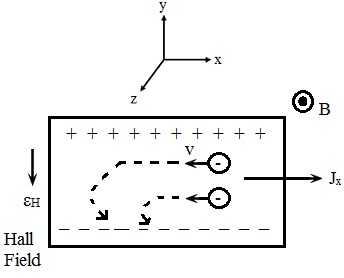Let us compute this Hall field. We begin from the Lorentz force acting on each and every electron F = -e [E+(v × B)]. According to [p(t + dt) - p(t)]/dt = dp/dt = F - p(t)/τ, we determine:

m (dv/dt) = -e [E + vxB) -m (v/τ)

Here 'τ' is the relaxation time. Note that the Lorentz force is not similar for all electrons as they move by different velocities; thus it is averaged over ensemble. We are searching for the solution of this equation in the steady state if the current is independent of time and thus dv/dt = 0.

0 = - eEx - eBvy - m(vx/τ)

0 = - eEy - eBvx - m(vy/τ)

We multiply the equations by - neτ/m to introduce the current densities components jx = -envx and jy = -envy, in such a way that,

σEx = ωcτjy + jx

σEy = - ωcτjx + jy

Here 'σ' is the Drude conductivity in the absence of the magnetic field. In steady state there is no electric current flowing perpendicular to the wire. Thus the Hall field EH = Ey can be found out by the need that there be no transverse current jy. Setting jy to zero in the second equation of the above equation we get:

Ey = -(ωcτ/σ)jx = -(1/ne)jxB

The proportionality constant -1/ne, is termed as the Hall constant, and is generally represented by RH. Thus,

RH = - (1/ne)

This is a extremely striking result that predicts that the Hall coefficient depends on no parameters of the metal apart from the density of carriers. As RH is inversely proportional to the electron concentration 'n', it follows that we can find out 'n' by measuring the Hall field. As we have already computed 'n' supposing that the atomic valence electrons become the metallic conduction electrons, a measurement of the Hall constant gives a direct test of the validity of this supposition.

Tutorsglobe: A way to secure high grade in your curriculum (Online Tutoring)

Expand your confidence, grow study skills and improve your grades.

Since 2009, Tutorsglobe has proactively helped millions of students to get better grades in school, college or university and score well in competitive tests with live, one-on-one online tutoring.

Using an advanced developed tutoring system providing little or no wait time, the students are connected on-demand with a tutor at www.tutorsglobe.com. Students work one-on-one, in real-time with a tutor, communicating and studying using a virtual whiteboard technology.  Scientific and mathematical notation, symbols, geometric figures, graphing and freehand drawing can be rendered quickly and easily in the advanced whiteboard.

Free to know our price and packages for online physics tutoring. Chat with us or submit request at info@tutorsglobe.com